# ISEE Upper Level Quantitative : Data Analysis

## Example Questions

### Example Question #61 : Data Analysis

Define a sequence as follows: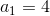For integers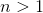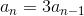.

Which is the greater quantity?

(a)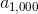(b)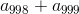It is impossible to tell which is greater from the information given

(a) is greater

(b) is greater

(a) and (b) are equal

(a) is greater

Explanation:

Subsituting: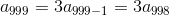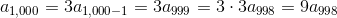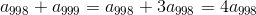Since the initial term of the sequence is positive, each subsequent term, 3 times the previous one, is positive, soThis makes (a) greater.

### Example Question #61 : Data Analysis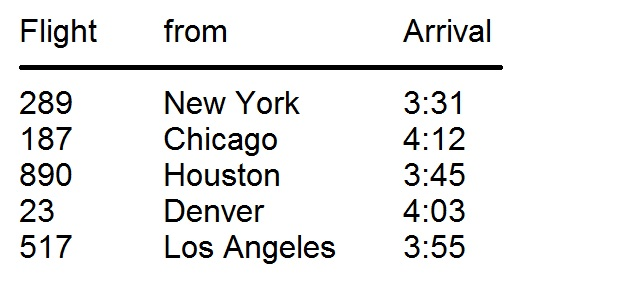Above is a part of a schedule of flight arrivals; the common destination is New Orleans, Lousiana, which is located in the Central Time Zone (CT). Chicago and Houston are also in the CT; New York is in the Eastern Time Zone, which is one hour ahead of CT; Denver is in the Mountain Time Zone, one hour behind CT; Los Angeles is in the Pacific Time Zone, two hours behind CT. All arrival times are PM and are given in Central Time.

When Flight 289 took off, it was 1:39 PM at its point of departure. How long did the flight take? (You may assume all flights arrived on time.)

1 hour, 52 minutes

3 hours, 8 minutes

2 hours, 52 minutes

2 hours, 8 minutes

3 hours, 52 minutes

2 hours, 52 minutes

Explanation:

Flight 289 departed from New York, which is in the Eastern Time Zone, one hour ahead of New Orleans. Since the flight took off when it was 1:39, subtract one hour; the flight took off when it was 12:39 in New Orleans. We need to subtract the adjusted departure time from the arrival time - however, this must be done by rewriting 12:39 as 0:39: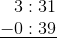"Borrow" one hour as follows: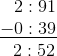The flight was 2 hours, 52 minutes long.

### Example Question #541 : Isee Upper Level (Grades 9 12) Quantitative ReasoningAbove is a part of a schedule of flight arrivals; the common destination is Atlanta, Georgia, which is located in the Eastern Time Zone. New York is also in the Eastern Time Zone; Chicago and Houston are in the Central Time Zone, which is one hour behind ET; Denver is in the Mountain Time Zone, two hours behind ET; Los Angeles is in the Pacific Time Zone, three hours behind ET. All arrival times are PM and are given in Eastern Time.

When Flight 23 took off, it was 11:21 AM at its point of departure. How long did the flight take? (You may assume all flights arrived on time.)

2 hours and 42 minutes

3 hours and 18 minutes

3 hours and 42 minutes

2 hours and 42 minutes

4 hours and 42 minutes

2 hours and 42 minutes

Explanation:

Flight 23 took off from Denver, which is in the Mountain Time Zone - two hours behind Atlanta time. Since it was 11:21 AM there, two hours must be added to this time to determine the time in Atlanta - this is 1:21 PM. Since the flight arrived in Atlanta at 4:03, the flight time was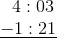We can "borrow" one hour, then subtract, as follows: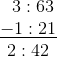The flight took 2 hours and 42 minutes.

### Example Question #541 : Isee Upper Level (Grades 9 12) Quantitative ReasoningAbove is a part of a schedule of flight arrivals; the common destination is Atlanta, Georgia, which is located in the Eastern Time Zone. New York is also in the Eastern Time Zone (ET); Chicago and Houston are in the Central Time Zone, which is one hour behind ET; Denver is in the Mountain Time Zone, two hours behind ET; Los Angeles is in the Pacific Time Zone, three hours behind ET. All arrival times are PM and are given in Eastern Time.

Flight 289 lasted 1 hour 57 minutes. When it took off, what time was it at the location of its departure?

11:34 AM

12:34 PM

1:34 PM

10:34 AM

2:34 PM

1:34 PM

Explanation:

Flight 289 took off from New York, which is in the same time zone as Atlanta, so no adjustments for Time Zone need to be made. Subtract 1 hour 57 minutes from the arrival time of 3:31: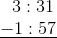We can "borrow" one hour and subtract as follows: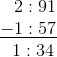It was 1:34 PM in New York when the airplane departed.

### Example Question #44 : Tables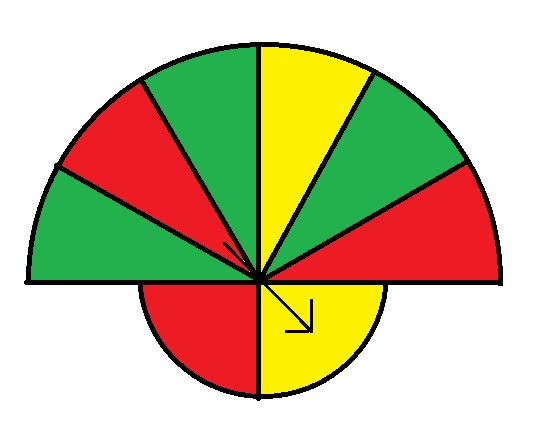The above figure shows a spinner used in a game. The upper semicircle has twice the radius of the lower semicircle.

Which of the following is the greater quantity?

(a) The probability that a random spin will cause the spinner to land in a green region.

(a) The probability that a random spin will cause the spinner to land in a red region.

It cannot be determined which of (a) and (b) is greater

(a) and (b) are equal

(a) is the greater quantity

(b) is the greater quantity

(b) is the greater quantity

Explanation:

The size of the regions do not matter in this problem; it is the central angles of the sectors that are relevant. The best way to understand this is to look at the spinner again, but after cutting out the central circle: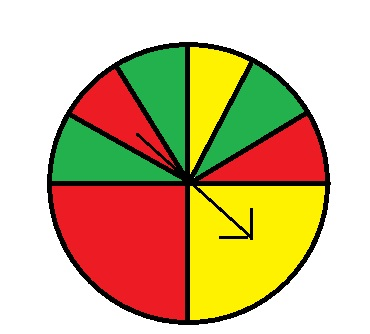If we rearrange the sectors, we see the following: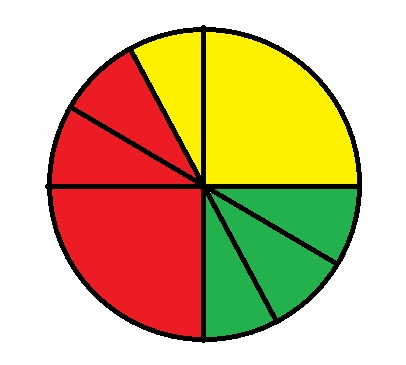The probability of the spinner landing in red exceeds that of it landing in green.

### Example Question #61 : Data Analysis

One ordinary six-sided die is altered such that the probability of tossing a six on that die is; a second such die is altered such that the probability of tossing a six on that die is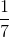. For each die, the other five rolls are equally probable to one another.

Which of the following is the greater quantity?

(a) The probability that the roll of two fair dice will come up a total of 12

(b) The probability that the roll of the two altered dice will come up a total of 12

It cannot be determined which of (a) and (b) is greater

(a) and (b) are equal

(b) is the greater quantity

(a) is the greater quantity

(b) is the greater quantity

Explanation:

The only way for a roll of two dice to come up with a sum of 12 is for the dice to show a double-six. Apply the multiplication principle using the probability of a roll of six on each die.

The probability of a roll of six on a fair die is, so the probability of a double-six happening with the fair dice is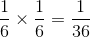.

The probability of a double-six on the altered dice is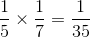.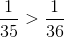, so (b) is greater.

### Example Question #51 : Tables

One ordinary six-sided die is altered such that the probability of tossing a six on that die is; a second such die is altered such that the probability of tossing a six on that die is. For each die, the other five rolls are equally probable to one another.

Which of the following is the greater quantity?

(a) The probability that the roll of two fair dice will come up a total of 2

(b) The probability that the roll of the two altered dice will come up a total of 2

(a) and (b) are equal

(b) is the greater quantity

It cannot be determined which of (a) and (b) is greater

(a) is the greater quantity

(a) is the greater quantity

Explanation:

There is only one way that the roll of two dice can come up 2 - for both dice to come up a one.

The probability of a fair die coming up a one is, so the probability of this happening with the fair dice is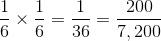.

The probability of a "1" coming up on the first altered die is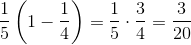The probability of a "1" coming up on the second altered die is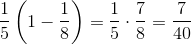Therefore, the probability of a roll of double one on the altered dice is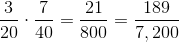Therefore, "2" is more likely to come up on the fair dice.

### Example Question #68 : Data Analysis And Probability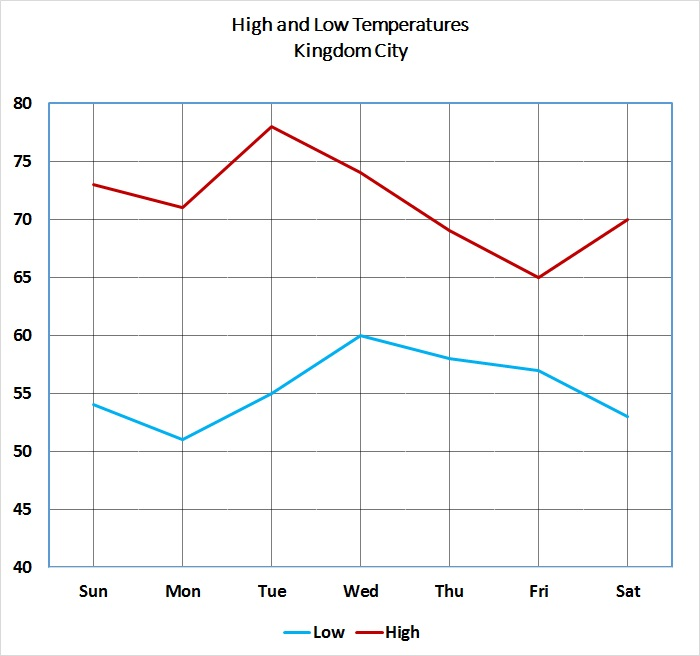Refer to the above graph, which shows the high and low temperatures for Kingdom City over a one-week period.

Which is the greater quantity?

(a) The temperature in Kingdom City at 6 AM Wednesday

(b) The temperature in Kingdom City at 6 PM Wednesday

(b) is the greater quantity

(a) and (b) are equal

It is impossible to determine which is greater from the information given

(a) is the greater quantity

It is impossible to determine which is greater from the information given

Explanation:

The line graph only indicates the high and low temperatures for the individual days; it says nothing about the temperature at any specific time of any day. Therefore, the question cannot be answered from the graph alone.

### Example Question #61 : Data AnalysisRefer to the above graph, which shows the high and low temperatures for Kingdom City over a one-week period.

Which is the greater quantity?

(a) The lowest high temperature for any of the seven days

(b) The highest low temperature for any of the seven days

(a) and (b) are equal

(b) is the greater quantity

It is impossible to determine which is greater from the information given

(a) is the greater quantity

(a) is the greater quantity

Explanation:

Examine the low point of the line representing the high temperatures and the high point of the line representing the low temperatures.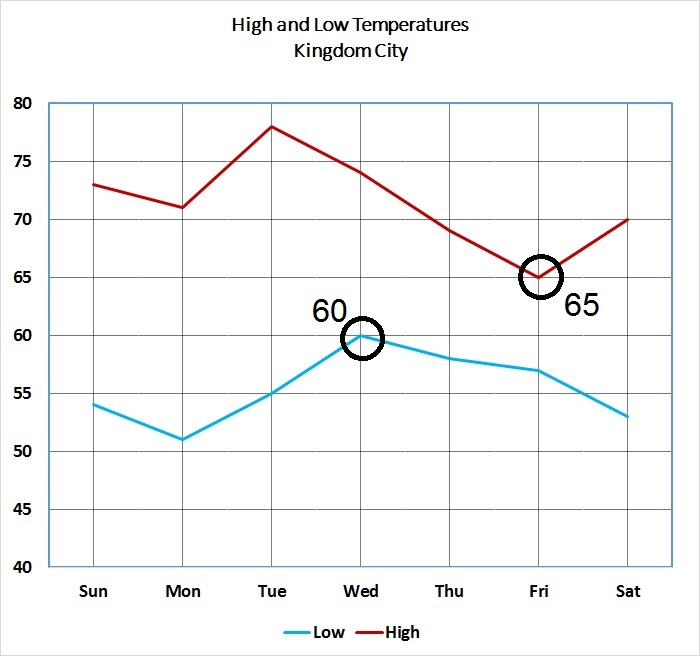It can be seen that the lowest of the high temperatures is 65 degrees (Friday), and that the highest of the low temperatures is 60 degrees (Wednesday). This makes the former quantity the greater.

### Example Question #70 : Data Analysis And ProbabilityRefer to the above graph, which shows the high and low temperatures in degrees Fahrenheit for Kingdom City over a one-week period.

Which is the greater quantity?

(a) The temperature in Kingdom City at noon on Friday

(b) 70 degrees

(b) is the greater quantity

(a) and (b) are equal

(a) is the greater quantity

It is impossible to determine which is greater from the information given

(b) is the greater quantity

Explanation:

The graph does not tell us the temperatures at any specific time of day on any of the seven days. However, as seen below, we know from the graph that the high temperature on Friday was 65 degrees.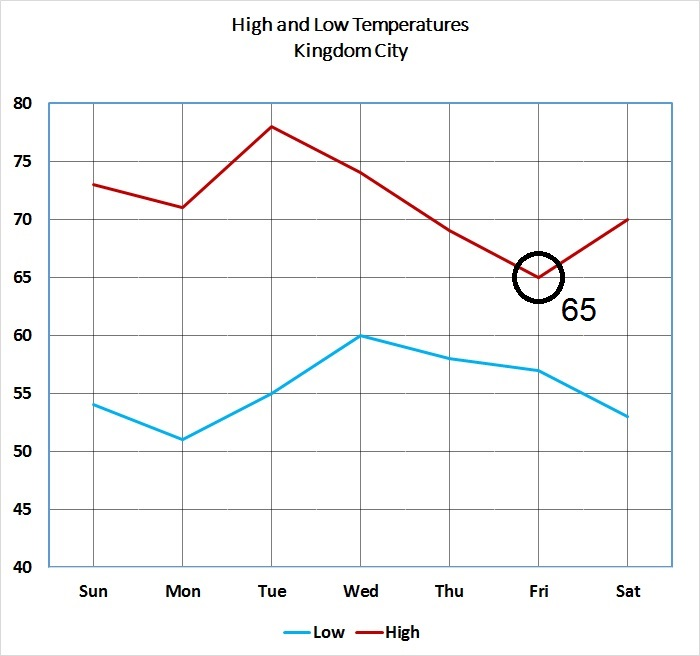The temperature at noon Friday cannot have been greater than 65 degrees, so 70 is the greater quantity.

### All ISEE Upper Level Quantitative Resources